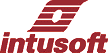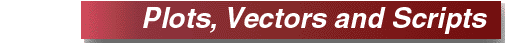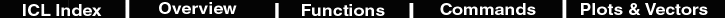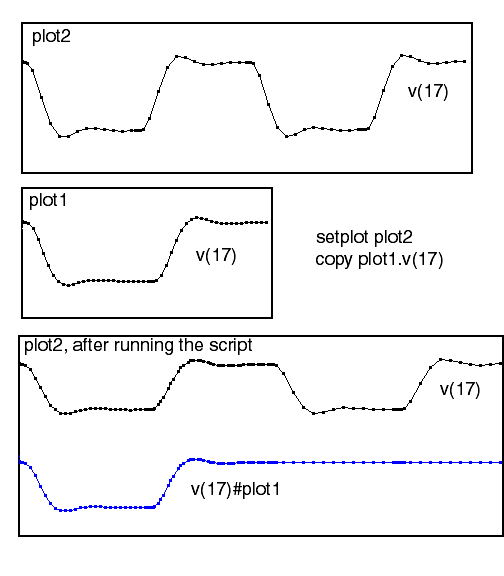During a simulation, Spice3 compatible simulators, including IsSpice4, organize simulation results into plots, one for each simulation that is run. The plots contain vectors (i.e. simulation waveforms and other results). Vectors are made for the node voltages and part parameters (current, power, etc.) that were identified using "save" commands. SpiceNet automatically makes "saves" for all test points, top level node voltages and most device currents and power dissipations. After a simulation is run, additional vectors are made to hold the results of different calculations; for example, the script: ```"vrms = rms(v(out))" produces a new vector called "vrms"., illustrated in the figure 1``` ```.control save v(out) save v(in) tran 1n 100n …```Figure 1. Both simulations and scripts create plot vectors. The plot name used here is called "tran1". The ICL script above does two saves for node voltages "out" and "in" and then runs a transient analysis.

 IsSpice4 scripts automatically cast variables to the correct type so that arithmetic can be performed on mixed types; that is, real, complex, scalar or vector quantities can be used with meaningful results. For example; when 2 vectors are multiplied, the corresponding elements of each vector are multiplied and placed in the resultant vector. The result is automatically adjusted to the correct type, even if it started off as something else. Intelligent type casting results in extremely powerful math operations taking place at speeds comparable to those achieved in the C/C++ programming language. Plots contain a special vector, called the default vector. The default vector is the independent variable or scale for a simulation - time for transient analysis and frequency for AC analysis. Plots from different simulations are likely to have different scales, if so, the vectors must be interpolated in order to combine their data. IsSpice4 provides the linearize script command to make the common scale uniformly spaced; an approach which is inaccurate if any data points have a separation in scale less than the linearized step. On the other hand, if the steps are made very small, the vectors use more storage than is necessary. IsSpice4 solves this problem by making a combined scale and interpolating data that needs to be manipulated into that new scale. This is accomplished with two additional commands that combine the scale of two plots such that each time point is preserved, These commands are "copy" and "newplot". "Copy" is illustrated in Figure 2.Figure 2. The copy command merges scales, making vector math possible.

 The copy command copies a vector from another plot to the current plot and re-scales all similar vectors in the current plot. The copied vector has a postfix added, "#plot", where "plot" is the source plot name. If the vector copied is the default, for example, "copy tran1.default" then no vector is copied, but a new scale is made and the vectors are interpolated to fit the new scale. The "newplot" command can be used to create a new plot initialized with all of the vectors from the parent plot and re-scaled combining the scale from another plot. For example "newplot temp tran1.default tran2.default" copies all of tran2 into temp and scales it by combining the tran1 and tran2 scales. In summary, plots are created to hold the results of a simulation. The save command identifies the vectors that the simulator will create. Operations between the vectors in different plots are accomplished by placing these vectors into plots that have the same default scale.